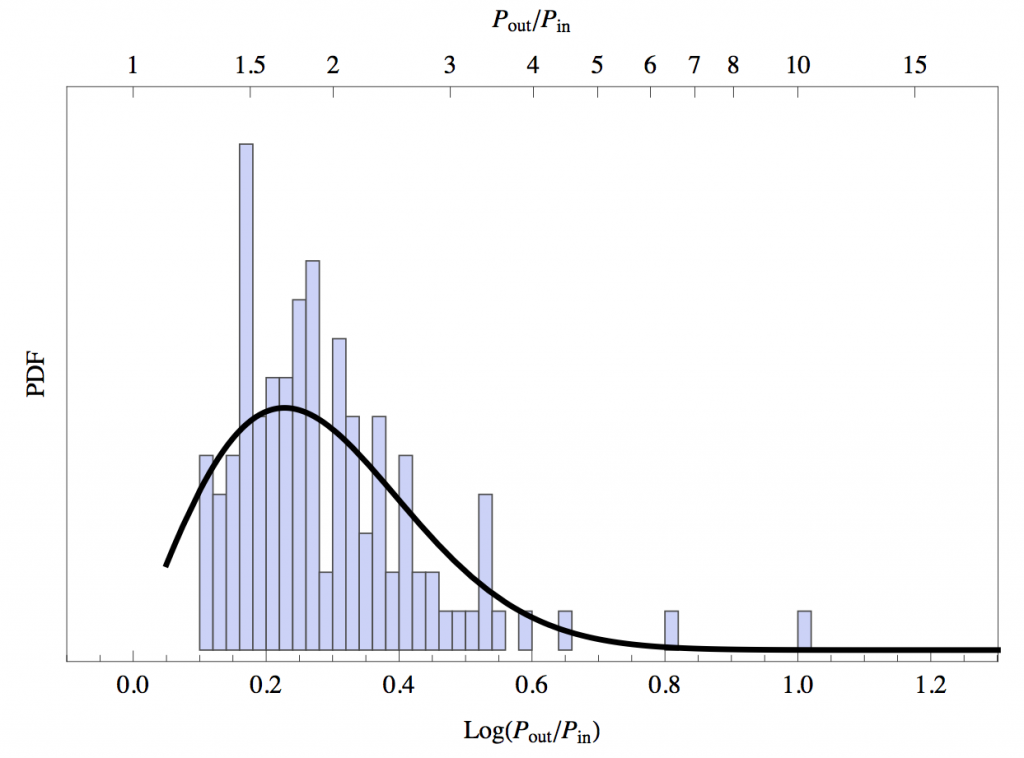# Hidden Siblings in Kepler Systems

Take a moment to pretend that you’re an alien observer looking at the Sun.  You can imagine that the light from the Sun will drop multiple times due to its eight transiting planets.  How often do stars within the Kepler database exhibit similar features?  In other words: how abundant are these multiplanet systems?  The short answer is very!  In fact, the Kepler mission is suggesting that thousands of such systems exist.

Any search through the exoplanet encyclopedia, however, will list multiple systems in which only two planets are known to transit.  But remember, in what I might dub the “Santa Claus Effect” – just because you can’t see it doesn’t mean it doesn’t exist.  Or to put it slightly more scientifically: it’s likely that these systems actually contain more unseen planets.  With multiple orbital inclinations among the planets it is plausible that some planets simply do not transit and may not be detected through Kepler photometry alone.  While orbital inclinations tend to vary by only a few degrees (making planetary systems relatively coplanar), even such a small variation in inclination may lead to unseen sibling planets.

Models of planet formation suggest that planetary systems are dynamically packed. As such they are stable and the ratios of orbital periods are generally not too large.  It is likely that two adjacent planets will have a mean motion resonance (what we will refer to as an orbital period ratio) of 3:2. In other words: one planet will orbit for three full periods while its neighbor will orbit for two full periods.  Certain orbital period ratios, such as 2:1, 3:2, and 5:2 are stable, in that these orbital period ratios remain as is. Steffen analyzes the distribution of orbital period ratios throughout the Kepler database. He does so for both high multiplicity systems and low multiplicity systems.

High Multiplicity SampleFigure 1: A histogram of the period ratios for the high multiplicity system.

All systems within this sample include four or more planets.  It contains 34 planetary systems with 146 planets, leaving 114 adjacent planet pairs to study. The first figure shows a histogram of the orbital period ratios for this system.  As expected, there is an excess of planets near the 3:2 (1.5) and 2:1 (2) orbital period ratio. Remember, both of these are stable.

There are also a few noticeably large gaps near the tail of the histogram.  These gaps could be due to missing planets, or a physical property of the protoplanetary disk.   If the gaps corresponded to a transition in the protoplanetary disk (such as a change in density) then one would expect that the two adjacent planets may have radically different sizes.  Steffen found that this was not the case – we do not see a large variance in the radii for large orbital period ratios. This outlaws the second hypothesis, and the gaps are likely due to missing planets.

Low Multiplicity System

All systems within this sample contain only two transiting planets.  It contains 244 systems, or 488 total planets.  The main hypothesis is that these systems are fundamentally high multiplicity systems, with two or more planets that simply do not transit.  Steffen reproduced the period ratio distribution of low multiplicity systems from the high multiplicity distribution.

There are the clear 3:2 and 2:1 orbital period ratios.  But there are two main discrepancies with the model.  The first is that there is also a slight excess in the low orbital period ratios, mainly between 2 and 3. Steffen looks at 4 possible explanations:Figure 3: Orbital period ratios in the low multiplicity sample. The two lines indicate the expected fit due to the high multiplicity system.  The dashed line does not include widely spaced planets.

(1) A statistical fluctuation – perhaps we simply need more data.

(2) There are missing planets – if we are only seeing the first and third planet then it is likely that we would observe an orbital period ratio between 2 and 3.

(3) Dynamical hypothesis – the dynamics of the 2:3, 3:1, and 5:2 orbital period ratios are so strong that planets have become trapped in their orbits.

(4) Kinematic hypothesis – At some point the orbits of two planets overlapped leading to the eventual collision of the two planets. This left a single planet in an orbit between the original two.  This does not seem unreasonable given how tightly packed these systems are.

While this excess is still not fully understood, answers may lead to considerable discoveries on the overall evolution of the system.  The second discrepancy may be seen in the gaps toward the high orbital period ratios. It was noted in the last figure that these gaps are not likely due to a transition in the protoplanetary disk but rather missing planets. Thus both discrepancies may be explained by missing planets.

Let’s look at our own solar system.  All of the orbital period ratios between adjacent planets are below 3:1, with one exception – the orbital period ratio of Mars/Jupiter clocks in at 6.3.  However, if we include Ceres (or the asteroid belt in general) than the gap disappears entirely and an orbital period ratio of 6.3 is replaced by a 2.4 and a 2.6.

Conclusions

If we study the general features of the low multiplicity system as predicted by the high multiplicity system then we may make statements about the formation and evolution of planetary systems as well as search for any additional planets.  It is likely that the two discrepancies are due to missing planets.  Nonetheless they will certainly help us with both theoretical studies concerning planetary systems as well as observational studies in finding further exoplanets.